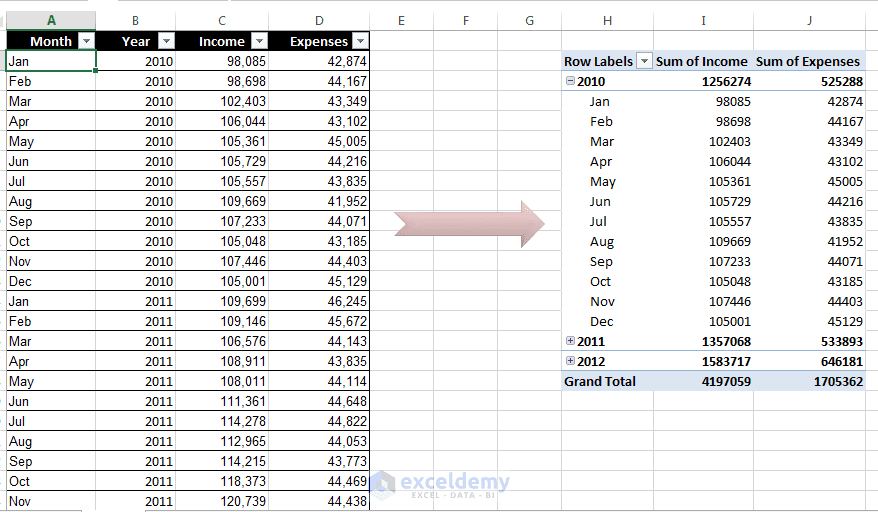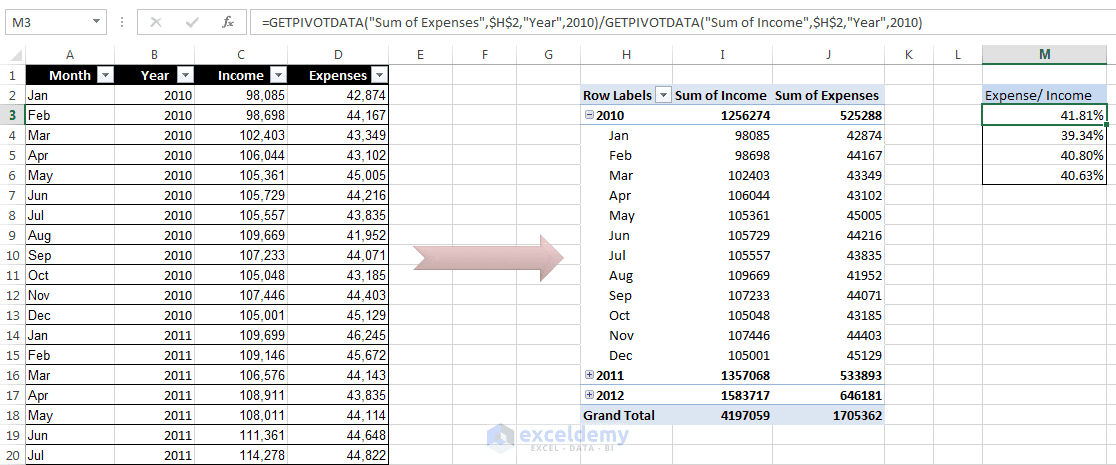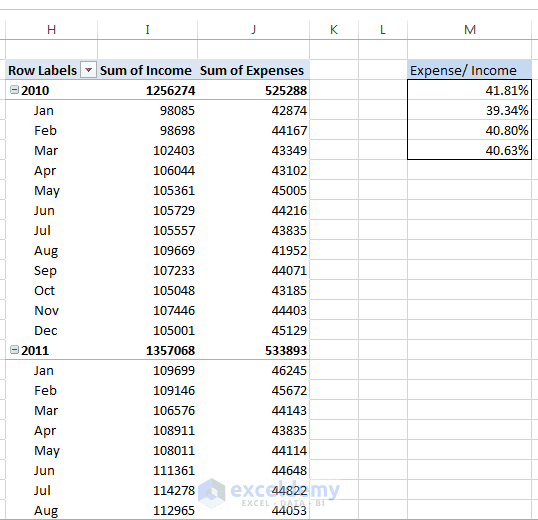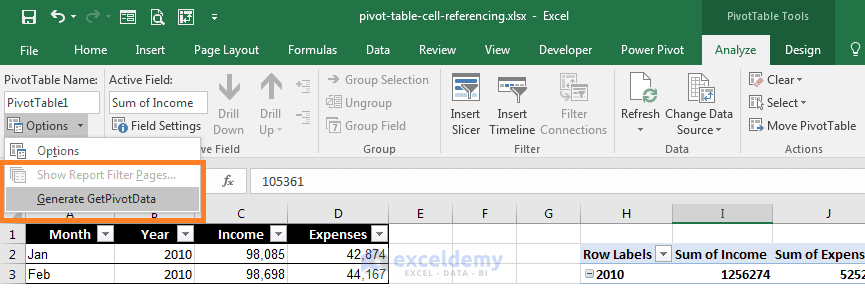Disclosure: This post may contain affiliate links, meaning when you click the links and make a purchase, we receive a commission.

# How to Reference PivotTable Data in Excel Formulas with GETPIVOTDATA?

In this article, I have shown you how you can reference PivotTable Cell Data in Excel formulas using GETPIVOTDATA function. If you feel uneasy with the auto implementation of GETPIVOTDATA function in the Excel formulas, you can stop it!

After you have created a pivot table, you may want to create formulas that will reference one or more cells within that pivot table. Here is the way how you can do that.

Related: Excel Pivot Table Tutorials for Dummies [Step by Step]

## Reference PivotTable Data Using GETPIVOTDATA Function

The following figure shows a pivot table. The pivot table displays income and expense information for the three years.A pivot table. We shall use this pivot table to explain cell references within this pivot table.

We entered a formula in column M, and this column is not part of our pivot table. Formulas entered into cells M3, M4, M5, and M6 will calculate the expense-to-income ratio for each year and grand total. To enter the formula in cell M3, we have selected cell M3 at first. Then we entered “=” sign, then selected cell J3, then entered “/” sign, and lastly selected cell I3. We should have “=J3/I3” in cell M3.

In fact, the formula in cell M3 is
=GETPIVOTDATA(“Sum of Expenses”,\$H\$2,”Year”,2010)/GETPIVOTDATA(“Sum of Income”,\$H\$2,”Year”,2010)

When we have used the pointing technique (selecting cells with the mouse when entering formula) to create a formula, Excel replaced those simple cell references with a much more complicated function GETPIVOTDATA. If you type the cell references manually (instead of pointing to them), Excel will not use the GETPIVOTDATA function. Using the GETPIVOTDATA function helps ensure that the formula will continue to reference the pointed cells if the pivot table layout is changed. The following figure shows the complete work we have done so far.Expense/ Income zone is calculating expense income ratio for three years and grand total.

The following shows the pivot table after expanding the years to show the month detail. You will see that the formulas in column M still show the accurate result even though the referenced cells in the formulas are in a different location. If I had used simple cell references like “=J3/I3”, the formula would not return the same result after expanding the years.The formula still shows the correct result after expanding the year.

Caution: Using the GETPIVOTDATA function has one limitation: The data that it retrieves must be visible. If you modify the pivot table so that the value used by GETPIVOTDATA is no longer visible, the formula will return an error.

Read More: Multiple Groups from the Same Data Source

## How to stop auto using of GETPIVOTDATA function?

If you want to prevent Excel from using the GETPIVOTDATA function when you point to pivot table cells at the time of creating a formula, choose PivotTable Tools ➪ Analyze ➪ PivotTable ➪ Options ➪ Generate GetPivot Data command. Deselect the check mark to turn off GETPIVOTDATA function working when you point cells in the pivot table. This command is a toggle.Stop auto using of GETPIVOTDATA function

1. Reply